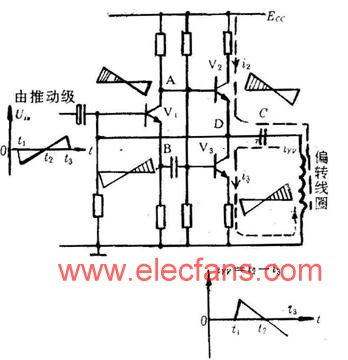# Principle Circuit and Principle Analysis of OTL Field Output Stage

The figure is the principle circuit of the OTL field output stage. Like the normal audio OTL circuit, it uses the two transistors V2 and V3 to supply the positive and negative portions of the scanning current iYV required for the deflection coil. The prescribed positive direction of iYV is the direction flowing into the deflection yoke in this figure. During the (t1 to t2) half cycle in which V2 is turned on, its current i2 flows into the coil through the DC blocking capacitor C to form the positive half of iYV. At this time, V3 is cut off, and i3=0. Capacitor C is charged by i2. When t=t2, iYV=0. During the half cycle of (t2~t3), V3 is turned on, V2 is turned off, the charge on the capacitor C is discharged through V3 and the coil, and the current i3 constitutes the negative half of iYV. The DC voltage at point D is ECC/2. V2 and V3 work in Class B or Class A and B. The place where they are handed over corresponds to the scanning forward center iYV=0. If the two tubes are not well connected or the magnification difference is large, the waveform of iYV at the junction is not a straight line, and the pattern at the center of the screen is distorted. Therefore, some feedback components need to be added in the actual circuit to make the output waveforms of the two tubes symmetrical.